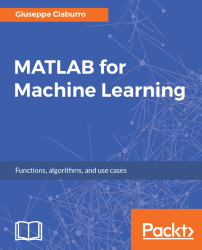•#### MATLAB for Machine Learning#### Overview of this book

MATLAB is the language of choice for many researchers and mathematics experts for machine learning. This book will help you build a foundation in machine learning using MATLAB for beginners. You’ll start by getting your system ready with t he MATLAB environment for machine learning and you’ll see how to easily interact with the Matlab workspace. We’ll then move on to data cleansing, mining and analyzing various data types in machine learning and you’ll see how to display data values on a plot. Next, you’ll get to know about the different types of regression techniques and how to apply them to your data using the MATLAB functions. You’ll understand the basic concepts of neural networks and perform data fitting, pattern recognition, and clustering analysis. Finally, you’ll explore feature selection and extraction techniques for dimensionality reduction for performance improvement. At the end of the book, you will learn to put it all together into real-world cases covering major machine learning algorithms and be comfortable in performing machine learning with MATLAB.
Title PageCreditsForewordwww.PacktPub.comCustomer FeedbackPrefaceFree Chapter
Getting Started with MATLAB Machine LearningImporting and Organizing Data in MATLABFrom Data to Knowledge DiscoveryFinding Relationships between Variables - Regression TechniquesPattern Recognition through Classification AlgorithmsIdentifying Groups of Data Using Clustering MethodsSimulation of Human Thinking - Artificial Neural NetworksImproving the Performance of the Machine Learning Model - Dimensionality ReductionMachine Learning in Practice## How to create a linear regression model

More generally, to create a linear regression model, use the `fitlm()` function. This function creates a `LinearModel` object. The object in the workspace has a series of properties that can be immediately viewed by simply clicking on it. Methods such as `plot`, `plotResiduals`, and `plotDiagnostics` are available if you want to create plots and perform a diagnostic analysis.

### Note

`LinearModel` is an object comprising training data, model description, diagnostic information, and fitted coefficients for a linear regression.

By default, `fitlm()` takes the last variable in the table or dataset array as the response. Otherwise, we have to specify predictors and response variables, for example, as a formula. In addition, we can set a specific column as the response variable by using the `ResponseVar` name-value pair argument. To use a set of the columns as predictors, use the `PredictorVars` name-value pair argument. Predictor variables can be numeric or of any grouping variable...